Next: Overlapping Sets of Sources Up: ENSEMBLES OF REARRANGEMENT PATHWAYS Previous: Graph Transformation Method   Contents

# Applications to Sparse Random Graphs

Algorithm B.3 could easily be adapted to use adjacency-lists-based data structures , resulting in a faster execution and lower storage requirements for sparse graphs. We have implemented  a sparse-optimised version of Algorithm B.3 because the graph representations of the Markov chains of interest in the present work are sparse .

The algorithm for detaching a single intermediate node from an arbitrary graph stored in a sparse-optimised format is given in Algorithm B.4. Having chosen the node to be removed,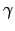, all the neighbours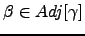are analysed in turn, as follows. Lines 3-9 of Algorithm B.4 find nodein the adjacency list of node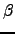. Ifis not a sink, lines 11-34 are executed to modify the adjacency list of node: lines 13-14 delete nodefrom the adjacency list of, while lines 15-30 make all the neighbours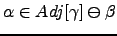of nodethe neighbours of. The symbol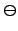denotes the union minus the intersection of two sets, otherwise known as the symmetric difference.If the edge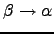already existed only the branching probability is changed (line 21). Otherwise, a new edge is created and the adjacency and branching probability lists are modified accordingly (line 26 and line 27, respectively). Finally, the branching probabilities of nodeare renormalised (lines 31-33) and the waiting time for nodeis increased (line 34).

Algorithm B.4 is invoked iteratively for every node that is neither a source nor a sink to yield a graph that is composed of source nodes and sink nodes only. Then the procedure described in Section 4.4 for disconnection of source nodes (lines 17-34 of Algorithm B.3) is applied to obtain the mean escape times for every source node. The sparse-optimised version of the second part of Algorithm B.3 is straightforward and is therefore omitted here for brevity.

The running time of Algorithm B.4 is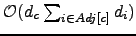, where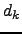is the degree of node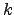. For the case when all the nodes in a graph have approximately the same degree,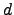, the complexity is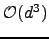. Therefore, if there are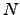intermediate nodes to be detached andis of the same order of magnitude as, the cost of detachingnodes is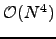. The asymptotic bound is worse than that of Algorithm B.3 because of the searches through adjacency lists (lines 3-9 and lines 19-24). Ifis sufficiently small the algorithm based on adjacency lists is faster.

After each invocation of Algorithm B.4 the number of nodes is always decreased by one. The number of edges, however, can increase or decrease depending on the in- and out-degree of the node to be removed and the connectivity of its neighbours. If nodeis not directly connected to any of the sinks, and the neighbours of nodeare not connected to each other directly, the total number of edges is increased by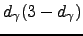. Therefore, the number of edges decreases (by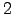) only when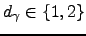, and the number of edges does not change if the degree is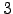. For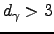the number of edges increases by an amount that grows quadratically with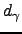. The actual increase depends on how many connections already existed between the neighbours of.

The order in which the intermediate nodes are detached does not change the final result and is unimportant if the graph is complete. For sparse graphs, however, the order can affect the running time significantly. If the degree distribution for successive graphs is sharp with the same average,, then the order in which the nodes are removed does not affect the complexity, which is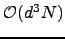. If the distributions are broad it is helpful to remove the nodes with smaller degrees first. A Fibonacci heap min-priority queue  was successfully used to achieve this result. The overhead for maintaining a heap isincrease-key operations (of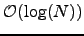each) per execution of Algorithm B.4. Fortran and Python implementations of Algorithm B.4 algorithm are available online .

Random graphs provide an ideal testbed for the GT algorithm by providing control over the graph density. A random graph,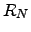, is obtained by starting with a set ofnodes and adding edges between them at random . In this work we used a random graph model where each edge is chosen independently with probability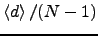, where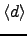is the target value for the average degree.

The complexity for removal ofnodes can then be expressed as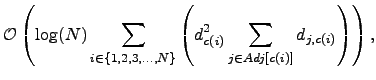(6.43)

where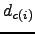is the degree of the node,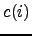, removed at iteration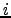,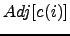is its adjacency list, and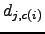is the degree of the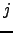th neighbour of that node at iteration. The computational cost given in Equation 4.43 is difficult to express in terms of the parameters of the original graph, as the cost of every cycle depends on the distribution of degrees, the evolution of which, in turn, is dependent on the connectivity of the original graph in a non-trivial manner (see Figure 4.10). The storage requirements of a sparse-optimised version of GT algorithm scale linearly with the number of edges.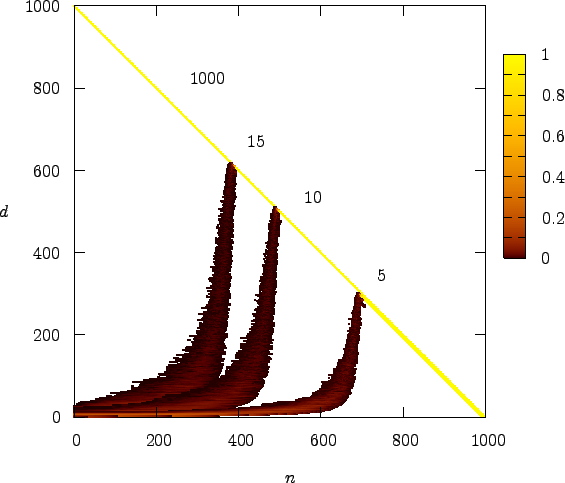To investigate the dependence of the cost of the GT method on the number of nodes,, we have tested it on a series of random graphsfor different values ofand fixed average degree,. The results for three different values ofare shown in Figure 4.11. The motivation for choosingfrom the interval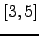was the fact that most of our stationary point databases have average connectivities for the local minima that fall into this range.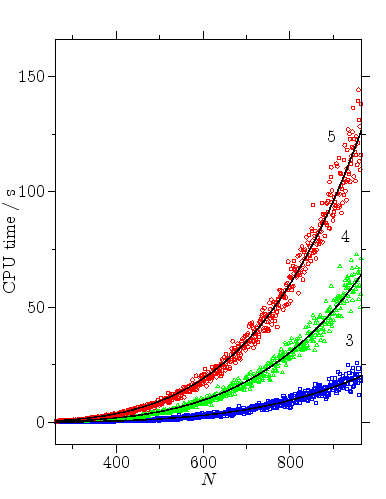It can be seen from Figure 4.11 that for sparse random graphsthe cost scales aswith a small-dependent prefactor. The dependence of the computational complexity onis illustrated in Figure 4.12.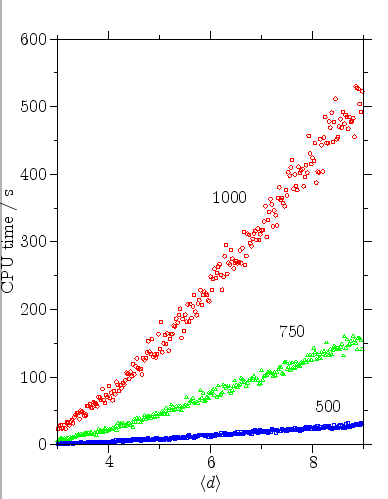From Figure 4.10 it is apparent that at some point during the execution of the GT algorithm the graph reaches its maximum possible density. Once the graph is close to complete it is no longer efficient to employ a sparse-optimised algorithm. The most efficient approach we have found for sparse graphs is to use the sparse-optimised GT algorithm until the graph is dense enough, and then switch to Algorithm B.3. We will refer to this approach as SDGT. The change of data structures constitutes a negligible fraction of the total execution time. Figure 4.13 depicts the dependence of the CPU time as a function of the switching parameter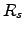.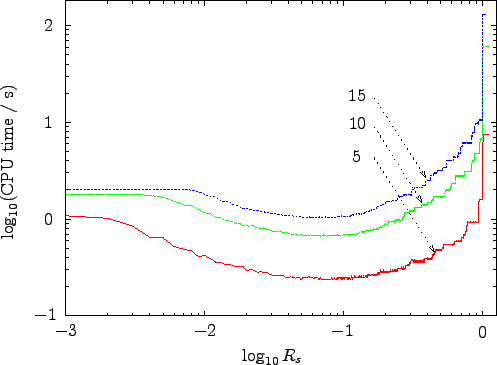Whenever the ratio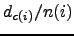, where theis the degree of intermediate node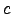detached at iteration, and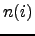is the number of the nodes on a heap at iteration, is greater than, the partially transformed graph is converted from the adjacency list format into adjacency matrix format and the transformation is continued using Algorithm B.3. It can be seen from Figure 4.10 that for the case of a random graphs with a single sink, a single source and 999 intermediate nodes the optimal values oflie in the interval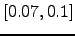.Next: Overlapping Sets of Sources Up: ENSEMBLES OF REARRANGEMENT PATHWAYS Previous: Graph Transformation Method   Contents
Semen A Trygubenko 2006-04-10